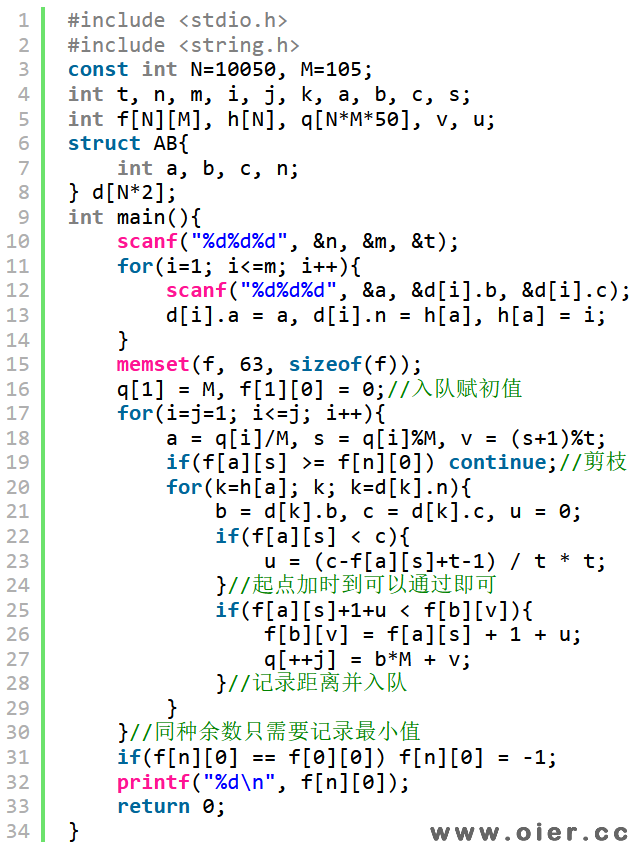311+

## 题目描述

“开放时间”$a _ i$，游客只有**不早于 $a _ i$ 时刻**才能通过这条道路。

## 输入输出样例

### 输入样例 #1

5 5 3
1 2 0
2 5 1
1 3 0
3 4 3
4 5 1

### 输出样例 #1

6

## 说明

**【样例 #1 解释】**

**【样例 #2】**

**【数据范围】**

| 测试点编号 | $n \leq$ | $m \leq$ | $k \leq$ | 特殊性质 |
|:-:|:-:|:-:|:-:|:-:|
| $1 \sim 2$ | $10$ |$15$ | $100$ | $a _ i = 0$ |
| $3 \sim 5$ | $10$ | $15$ | $100$ | 无 |
| $6 \sim 7$ | $10 ^ 4$ | $2 \times 10 ^ 4$ | $1$ | $a _ i = 0$ |
| $8 \sim 10$ | $10 ^ 4$ | $2 \times 10 ^ 4$ | $1$ | 无 |
| $11 \sim 13$ | $10 ^ 4$ | $2 \times 10 ^ 4$ | $100$ | $a _ i = 0$ |
| $14 \sim 15$ | $10 ^ 4$ | $2 \times 10 ^ 4$ | $100$ | $u _ i \leq v _ i$ |
| $16 \sim 20$ | $10 ^ 4$ | $2 \times 10 ^ 4$ | $100$ | 无 |

## 解题思路

（不能等待1秒，但是可以等待t秒，因为可以晚出发t秒！）

## 程序实现